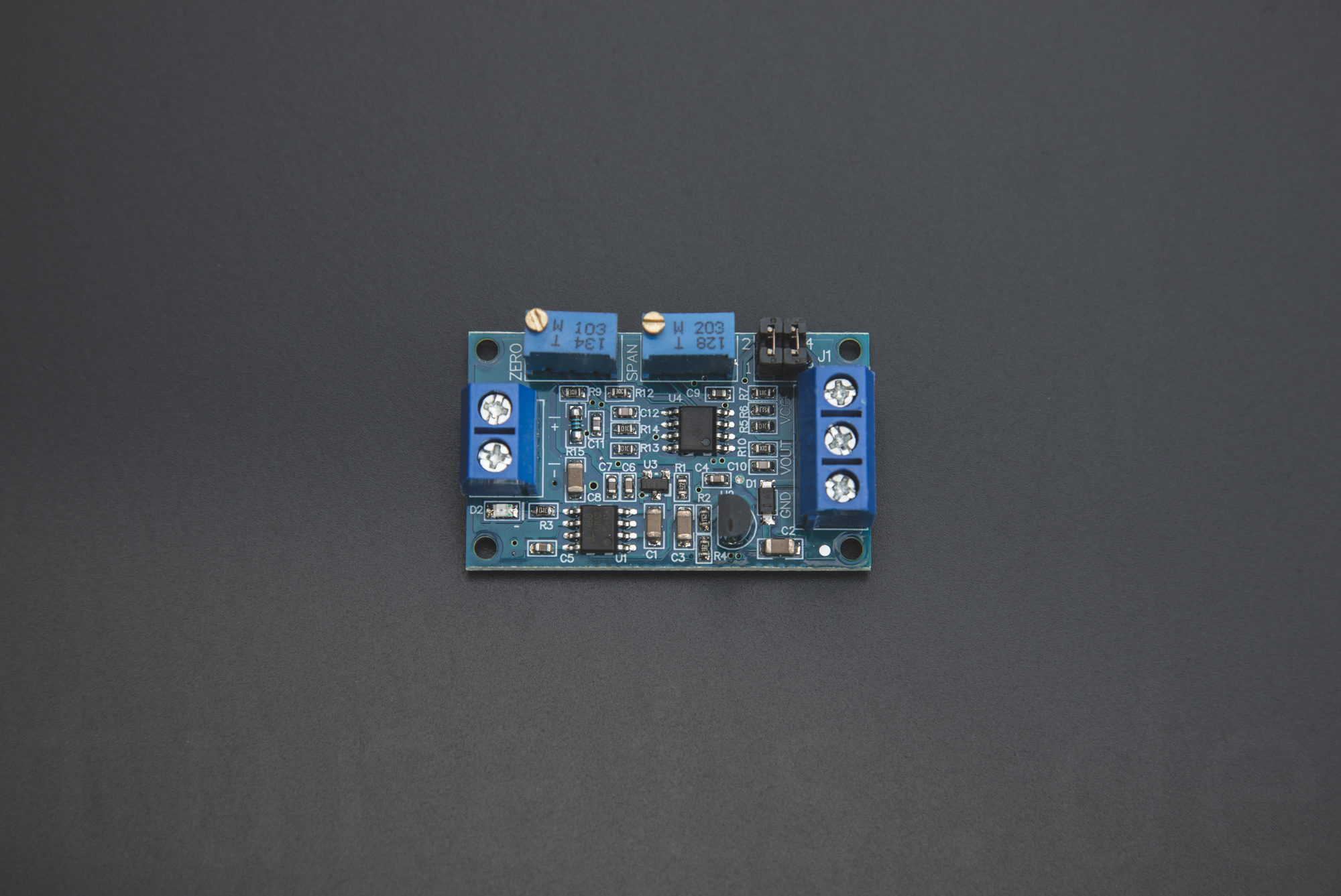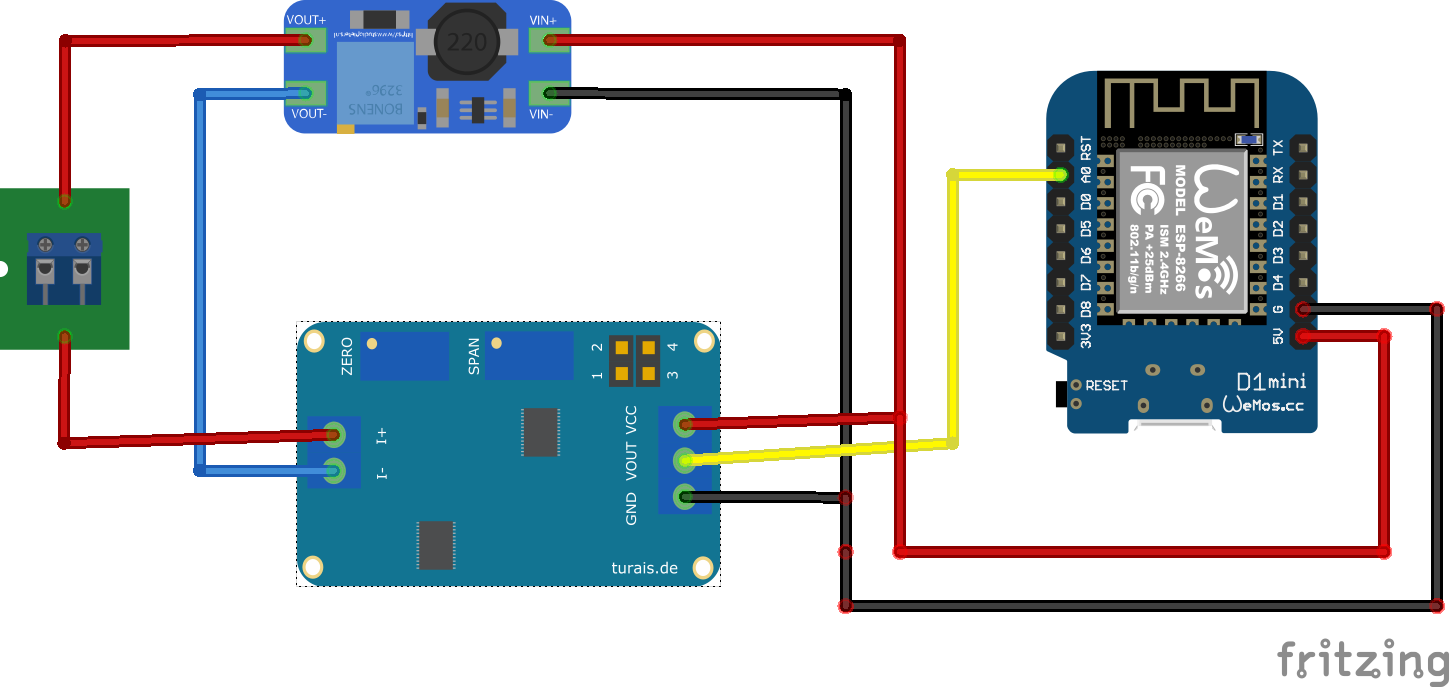# HW-685 4-20mA Current Sensing

The HW-685 4-20mA Current Sensing Module finds its place in a situation you want to measure small currents from 4 to 20mA. It is fairly cheap, about 1-2\$.

## OverviewThe HW-685 4-20mA Current Sensing Module

With this module you can measure currents from 4-20mA. It ‘translates’ the measured current into voltage. With two jumpers you can define the voltage output range. Also the module has two potentiometers for calibrating the zero and maximum voltage.

## ConnectionExample connection of the HW-685 module.

For a connection you need a constant voltage power supply. Connect the module like so:

Wemos D1 Mini PIN HD-685 Remarks
5V VCC 5V - 12V constant
G GND Ground
HD-685 Remarks
VCC 5V - 12V constant
GND Ground
I+ Positive Current In, after your sensor.
I- Current out. Usually you connect this to GND.

## Usage

Before you can measure reliable results, you need to calibrate your module with the two potentiometers. For this you need a constant current. I used a trim-potentiometer with an multimeter in current sensing mode, this is not optimal but I did not have any other method at hand. You can also use a dedicated “Signal Generator”. They are fairly cheap on Aliexpress or Amazon.

### Setting the Output Voltage

First you have to set the desired maximum output voltage for your `VOUT`. Most microcontrollers don’t ‘like’ more than 5V, be sure to check that out. For example if you use an Arduino it is okay to use 5V as your maximum voltage for your ADC.

Voltage Range Jumper Setting Remarks
0 - 3.3V `[ _ | _ ]` “1-2” empty, “3-4” empty.
0 - 5V `[ X | _ ]` “1-2” connected, “3-4” empty.
0 - 10V `[ X | X ]` “1-2” connected, “3-4” connected.

### Calibrating the Module

1. Turn all potentiometers to the left. You’ll hear a little click if the leftmost position is reached. This may take a while.

2. Connect you constant voltage power supply to `VCC` and a Multimeter to `VOUT`.

3. Connect your 4mA current source in series to `I+` and `I-`.

4. Now turn the `ZERO` potentiometer to the right, until you reach a voltage you will later define as the 4mA point.

5. Change the current source to 20mA and turn the `SPAN` potentiometer to a voltage you will later define as the 20mA point.

For example with an Arduino you have an 10-Bit ADC. This means that the incoming voltage of your ADC input is divided in intervals. If you have an 10-Bit ADC these intervals will be 2^10 = 1024 values between 0 and 1024, therefore you have 1023 intervals.

``````int analogPin = A3;
float ref_voltage = 3.3;

void setup() {
Serial.begin(9600);
}

void loop() {
}
``````

## Code

### Libraries

No Libraries needed.

### Example

``````#include <Arduino.h>

int analogPin = A0;
float max_curr_ma = 20.0;

void setup() {
// put your setup code here, to run once:
Serial.begin(9600);
}

void loop() {
// put your main code here, to run repeatedly:
}
``````

#### Example on Github

You can find the GitHub example here.

#### Blynk Example

``````#include <Arduino.h>
#define BLYNK_PRINT Serial
#include <ESP8266WiFi.h>
#include <BlynkSimpleEsp8266.h>

char auth[] = "YourAuthToken";
char ssid[] = "YourNetworkName";

int analogPin = A0;
float max_curr_ma = 20.0;

BlynkTimer timer;

void timer_event() {
}

void setup() {
Serial.begin(9600);
Blynk.begin(auth, ssid, pass);
//Blynk.begin(auth, ssid, pass, "blynk-cloud.com", 80);
timer.setInterval(1000L, timer_event);
}

void loop() {
Blynk.run();
timer.run();
}
``````